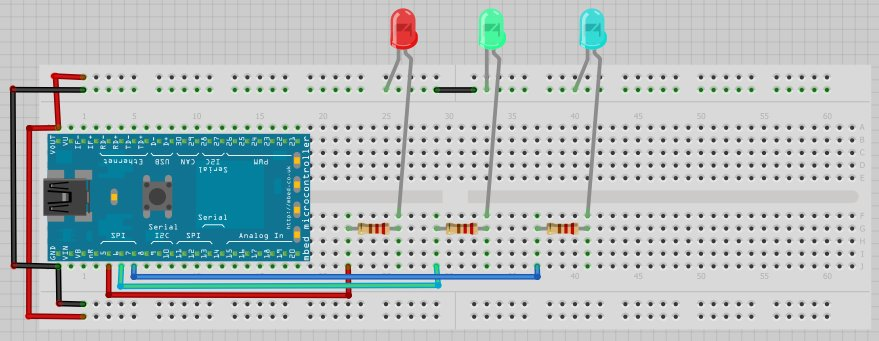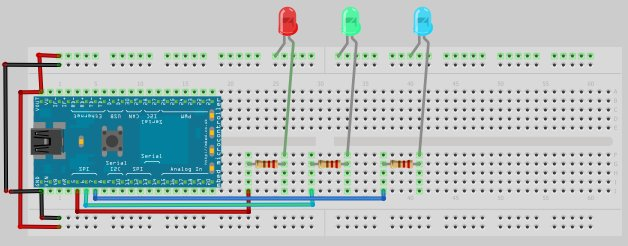## Digital Output

### Example 1

1. The program blinks the onboard LED 1 at 1/0.2 = 5 Hz.
2. `DigitalOut` is a C++ class. `myled` is declared as an object of `DigitalOut` using pin `LED`.
3. `wait(0.2)` cause the microcontroller busy-waiting for next statement to be executed.
4. `while ( )` means "while the statement within the bracket is `true`'". In C/C++, anything but `0` is `true`. So the statement `while (1)` is always evaluated to be true and hence it runs non-stop. You may like to substitute `-1`, `100`, `0`, `'0'`, `"0"`, `"Hi"` to test out.
```#include "mbed.h"

DigitalOut myled(LED1);

int main() {
while (1) {
myled = 1;
wait(0.2);
myled = 0;
wait(0.2);
}
}
```

#### Note

1. Another way to code the program above.
2. `!` means logical not.
```#include "mbed.h"

DigitalOut myled(LED1);

int main() {
myled = 1;
while (1) {
myled = !myled;
wait(0.2);
}
}
```

### Example 2

1. The program blinks the onboard LED 1 and LED 2 at 1/0.2 = 5 Hz, alternatively.
2. As we need to distinguish the LEDs from each other, we have to name them differently. In C/C++, no two variables share the same name. Name is case sensitive and may consist of letters, digits, and underscores, but they must not begin with a digit.
```#include "mbed.h"

DigitalOut myled1(LED1);
DigitalOut myled2(LED2);

int main() {
myled1 = 1;
myled2 = 0;
while (1) {
myled1 = !myled1;
myled2 = !myled2;
wait(0.2);
}
}
```

#### Note

1. The program can be written using member functions of `DigitalOut`.
```#include "mbed.h"

DigitalOut myled1(LED1);
DigitalOut myled2(LED2);

int main() {
myled1.write(1);
myled2.write(0);
while (1) {
wait(0.2);
}
}
```

### Example 3

1. The program passes a light from LED 1 to LED4, cyclically.
2. An array of `DigitalOut` objects was declared and initialized at same time. The definition of `DigitalOut` requires us to define the pin during its variable declaration. Look at the constructor for `DigitalOut`.
3. To access individual object in the array, we use a numerical index, starting from 0. The index is expressed with square brackets `[]`.
4. `for (i = 0; i < 4; i++)` setups a looping mechanism which initially setup the value of `i` to 0. Before the body of the `for` loop is executed, the statement `i < 4` is evaluated. If it is true, the loop body is executed. After each iteration, `i` is increment by 1.
```#include "mbed.h"

DigitalOut myleds[] = {(LED1), (LED2), (LED3), (LED4)};

int main() {
int i;
int previous;

while (1) {
for (i = 0; i < 4; i++) {
if (i == 0)
previous = 3;
else
previous = i - 1;

myleds[i] = 1;
myleds[previous] = 0;

wait(0.2);
}
}
}
```

#### Note

1. The program above can be written as follows.
2. `%` means "get the remainder after divided by".
3. `++i` means "increment i by 1 first before doing anything else".
4. The code is shorter but is more cryptic.
```#include "mbed.h"

DigitalOut myleds[] = {(LED1), (LED2), (LED3), (LED4)};

int main() {
int i = 0;
int previous = 3;

while (1) {
myleds[i] = 1;
myleds[previous] = 0;
previous = i;
i = ++i % 4;
wait(0.2);
}
}
```

### Example 4

1. The program below blinks 3 external LEDs which are red, green and blue.

#### Note

A LED has positive (anode) and negative (cathode) ends. A new LED has its positive end on the longer lead. You can also use digital multimeter to determine its polarity.#### Note

To prevent a high current passes through the LED and hence damaging it, it is usually connected with a resistor in series. For this example, we will use a 220 Ω resistor.

LEDPositive EndNegative End
Red`p5``GND`
Green`p6``GND`
Blue`p7``GND`
```#include "mbed.h"

DigitalOut red  (p5);
DigitalOut green(p6);
DigitalOut blue (p7);

int main() {
red = 1;
green = blue = 0;
wait(0.2);

green = 1;
red = blue = 0;
wait(0.2);

blue = 1;
red = green = 0;
wait(0.2);
}
```

Discovery

1. Use digital multimeter to measure the voltage
1. between each DigitalOut pin (p5 or p6 or p7) and the ground.
2. between the 220 Ω resistor.
3. between each LED.
2. Compare your results with the information from http://www.oksolar.com/led/led_color_chart.htm

Questions

1. Determine the voltage across each of the LED.
2. Determine the current passes through each of the LED.

### Example 5

1. The program below blinks 3 external LEDs which are red, green and blue. The connection is different from Example 4.LEDPositive EndNegative End
Red`VOUT``p5`
Green`VOUT``p6`
Blue`VOUT``p7`
```#include "mbed.h"

DigitalOut red  (p5);
DigitalOut green(p6);
DigitalOut blue (p7);

int main() {
red = 0;
green = blue = 1;
wait(0.2);

green = 0;
red = blue = 1;
wait(0.2);

blue = 0;
red = green = 1;
wait(0.2);
}
```